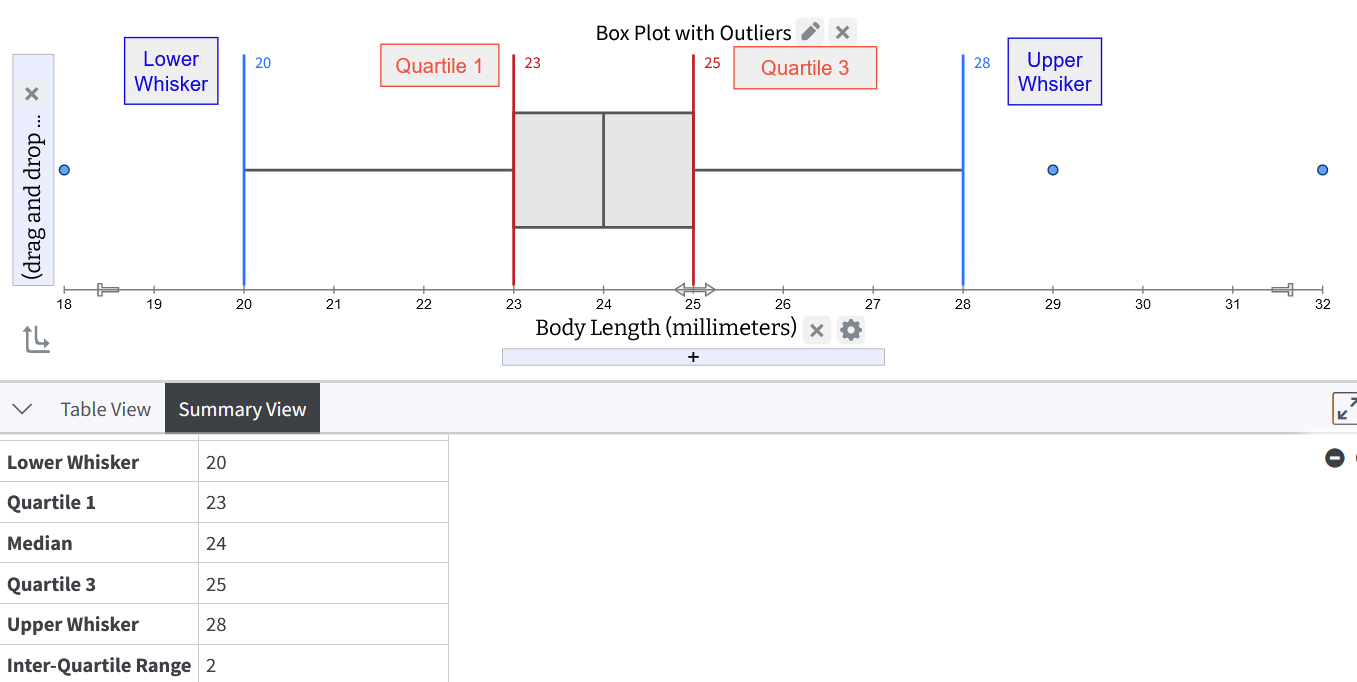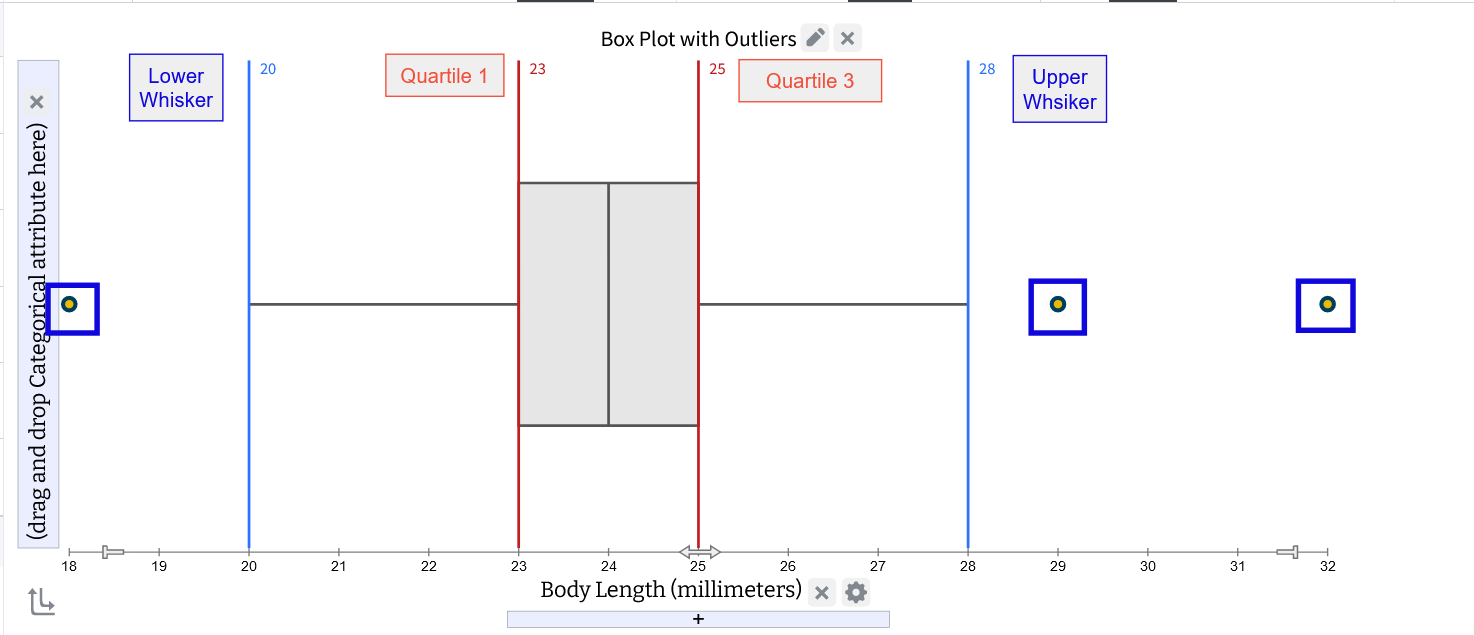# Computing Outliers in Tuva

The identification of outliers plays an important role in statistical analyses, aiding in the detection of exceptional data points. It is critical to identify outliers because they may be the “exception that proves the rule”, or they may be, upon closer examination, measurement error or fraudulent data that should be questioned as to its accuracy. This article delves into the underlying rule employed by Tuva to compute outliers for boxplots.

Within Tuva, users have the option to generate boxplots that incorporate outliers. The software leverages Tukey's rule, a fundamental guideline established by John Tukey, to determine these outliers. The rule centers around the quartiles of the dataset, dividing it into four equal parts.

Here is an example from the Cicadas dataset.:The interquartile range (IQR) is calculated as the difference between Quartile 3 and Quartile 1 (IQR = Q3 - Q1), offering a measure of the data's spread or dispersion within the middle range. In the above example, the IQR is = 25 - 23, that is equal to 2.

Application of Tukey’s Thumb Rule: Tuva employs Tukey's rule to identify outliers by establishing a "fence" beyond the first quartile (Q1) and the third quartile (Q3). Any values that fall outside this fence are recognized as outliers.

The software constructs this fence by calculating 1.5 times the interquartile range (IQR) and then subtracting this value from Q1 (Q1 - 1.5 * IQR) and adding it to Q3 (Q3 + 1.5 * IQR).

The lower whisker value is then increased until it coincides with the next-largest data value ( >= Q1 - 1.5 * IQR), and the upper whisker value is decreased until it coincides with the next-lowest data value (<= Q3 - 1.5 * IQR).

These resulting values serve as the minimum and maximum fence posts against which each data point is compared. Consequently, any values that exceed 1.5 times the IQR below Q1 or above 1.5 times the IQR above Q3 are identified as outliers.  If no values are beyond these fence posts, they are identical to the data minimum and maximum values.

In the case of the Body Length attribute in the Cicadas dataset, we find that 1.5 times the IQR is 1.5 multiplied by 2, resulting in 3.

Therefore, the lower fence is positioned at a value of 20 (Q1 - 1.5 * IQR), and the upper fence is located at 28 (Q3 + 1.5 * IQR). There are data points at 20 and 28, so the whiskers do not need to be adjusted further.

Any value in the dataset that falls below 20 or exceeds 28 would be classified as an outlier according to these fence boundaries.Why only 1.5 times the IQR? The number 1.5 is the "scale" that determines how sensitive we are to detecting outliers. A larger scale means that outliers are less likely to be identified, and they may be treated as regular data points. On the other hand, a smaller scale increases the chances of some normal data points being labeled as outliers. When we use a scale of 1.5 in the interquartile range (IQR) method, it means that any data point lying more than 2.7 standard deviations (σ) away from the mean (μ), in either direction, will be considered an outlier. This range closely aligns with what a Normal distribution (a bell-shaped curve) suggests, which is a range of 3 standard deviations for outlier detection.